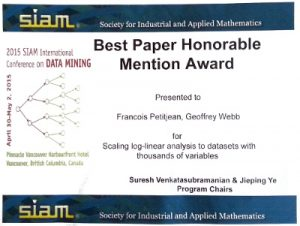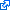# Scalable Graphical ModelingGraphical models are powerful descriptions of joint probability distributions. We have developed techniques for efficiently scaling exact methods to thousands of variables.

Check out the promotional video for our KDD-2106 paper:

Check out our interactive visualisation of the network Chordalysis learns about associated stock movements within the S&P500:### Publications

Petitjean, F., & Webb, G. I.
Proceedings of the ACM SIGKDD Conference on Knowledge Discovery and Data Mining, KDD-16, pp. 2131-2132, 2016.[Bibtex]

``````@InProceedings{PetitjeanWebbTut16,
Title = {Scalable Learning of Graphical Models},
Author = {F. Petitjean and G.I. Webb},
Booktitle = {Proceedings of the ACM SIGKDD Conference on Knowledge Discovery and Data Mining, KDD-16},
Year = {2016},
Pages = {2131-2132},
Publisher = {ACM Press},
Keywords = {scalable graphical models and Learning from large datasets and DP140100087},
Related = {scalable-graphical-modeling},
Url = {http://dl.acm.org/authorize?N19101}
}``````
``ABSTRACT ``

Webb, G. I., & Petitjean, F.
Proceedings of the ACM SIGKDD Conference on Knowledge Discovery and Data Mining, KDD-16, pp. 1255-1264, 2016.[Bibtex] [Abstract]

``````@InProceedings{WebbPetitjean16,
author = {Webb, Geoffrey I. and Petitjean, Francois},
booktitle = {Proceedings of the ACM SIGKDD Conference on Knowledge Discovery and Data Mining, KDD-16},
title = {A multiple test correction for streams and cascades of statistical hypothesis tests},
year = {2016},
pages = {1255-1264},
publisher = {ACM Press},
abstract = {Statistical hypothesis testing is a popular and powerful tool for inferring knowledge from data. For every such test performed, there is always a non-zero probability of making a false discovery, i.e.~rejecting a null hypothesis in error. Familywise error rate (FWER) is the probability of making at least one false discovery during an inference process. The expected FWER grows exponentially with the number of hypothesis tests that are performed, almost guaranteeing that an error will be committed if the number of tests is big enough and the risk is not managed; a problem known as the multiple testing problem. State-of-the-art methods for controlling FWER in multiple comparison settings require that the set of hypotheses be pre-determined. This greatly hinders statistical testing for many modern applications of statistical inference, such as model selection, because neither the set of hypotheses that will be tested, nor even the number of hypotheses, can be known in advance.
This paper introduces Subfamilywise Multiple Testing, a multiple-testing correction that can be used in applications for which there are repeated pools of null hypotheses from each of which a single null hypothesis is to be rejected and neither the specific hypotheses nor their number are known until the final rejection decision is completed.
To demonstrate the importance and relevance of this work to current machine learning problems, we further refine the theory to the problem of model selection and show how to use Subfamilywise Multiple Testing for learning graphical models.
We assess its ability to discover graphical models on more than 7,000 datasets, studying the ability of Subfamilywise Multiple Testing to outperform the state of the art on data with varying size and dimensionality, as well as with varying density and power of the present correlations. Subfamilywise Multiple Testing provides a significant improvement in statistical efficiency, often requiring only half as much data to discover the same model, while strictly controlling FWER.},
comment = {Top reviewer score (4.75/5.0), shortlisted for best paper award and invited to ACM TKDE journal KDD-16 special issue},
doi = {10.1145/2939672.2939775},
keywords = {Association Rule Discovery and statistically sound discovery and scalable graphical models and Learning from large datasets and DP140100087},
related = {statistically-sound-association-discovery},
url = {http://dl.acm.org/authorize?N19100},
}``````
``ABSTRACT Statistical hypothesis testing is a popular and powerful tool for inferring knowledge from data. For every such test performed, there is always a non-zero probability of making a false discovery, i.e.~rejecting a null hypothesis in error. Familywise error rate (FWER) is the probability of making at least one false discovery during an inference process. The expected FWER grows exponentially with the number of hypothesis tests that are performed, almost guaranteeing that an error will be committed if the number of tests is big enough and the risk is not managed; a problem known as the multiple testing problem. State-of-the-art methods for controlling FWER in multiple comparison settings require that the set of hypotheses be pre-determined. This greatly hinders statistical testing for many modern applications of statistical inference, such as model selection, because neither the set of hypotheses that will be tested, nor even the number of hypotheses, can be known in advance. This paper introduces Subfamilywise Multiple Testing, a multiple-testing correction that can be used in applications for which there are repeated pools of null hypotheses from each of which a single null hypothesis is to be rejected and neither the specific hypotheses nor their number are known until the final rejection decision is completed. To demonstrate the importance and relevance of this work to current machine learning problems, we further refine the theory to the problem of model selection and show how to use Subfamilywise Multiple Testing for learning graphical models. We assess its ability to discover graphical models on more than 7,000 datasets, studying the ability of Subfamilywise Multiple Testing to outperform the state of the art on data with varying size and dimensionality, as well as with varying density and power of the present correlations. Subfamilywise Multiple Testing provides a significant improvement in statistical efficiency, often requiring only half as much data to discover the same model, while strictly controlling FWER.``

Petitjean, F., & Webb, G. I.
Proceedings of the 2015 SIAM International Conference on Data Mining, pp. 469-477, 2015.[Bibtex] [Abstract]

``````@InProceedings{PetitjeanWebb15,
Title = {Scaling log-linear analysis to datasets with thousands of variables},
Author = {F. Petitjean and G.I. Webb},
Booktitle = {Proceedings of the 2015 {SIAM} International Conference on Data Mining},
Year = {2015},
Pages = {469-477},
Abstract = {Association discovery is a fundamental data mining task. The primary statistical approach to association discovery between variables is log-linear analysis. Classical approaches to log-linear analysis do not scale beyond about ten variables. We have recently shown that, if we ensure that the graph supporting the log-linear model is chordal, log-linear analysis can be applied to datasets with hundreds of variables without sacrificing the statistical soundness . However, further scalability remained limited, because state-of-the-art techniques have to examine every edge at every step of the search. This paper makes the following contributions: 1) we prove that only a very small subset of edges has to be considered at each step of the search; 2) we demonstrate how to efficiently find this subset of edges and 3) we show how to efficiently keep track of the best edges to be subsequently added to the initial model. Our experiments, carried out on real datasets with up to 2000 variables, show that our contributions make it possible to gain about 4 orders of magnitude, making log-linear analysis of datasets with thousands of variables possible in seconds instead of days.},
Comment = {Best Research Paper Honorable Mention Award},
Keywords = {Association Rule Discovery and statistically sound discovery and scalable graphical models and Learning from large datasets and DP140100087},
Related = {scalable-graphical-modeling},
Url = {http://epubs.siam.org/doi/pdf/10.1137/1.9781611974010.53}
}``````
``ABSTRACT Association discovery is a fundamental data mining task. The primary statistical approach to association discovery between variables is log-linear analysis. Classical approaches to log-linear analysis do not scale beyond about ten variables. We have recently shown that, if we ensure that the graph supporting the log-linear model is chordal, log-linear analysis can be applied to datasets with hundreds of variables without sacrificing the statistical soundness . However, further scalability remained limited, because state-of-the-art techniques have to examine every edge at every step of the search. This paper makes the following contributions: 1) we prove that only a very small subset of edges has to be considered at each step of the search; 2) we demonstrate how to efficiently find this subset of edges and 3) we show how to efficiently keep track of the best edges to be subsequently added to the initial model. Our experiments, carried out on real datasets with up to 2000 variables, show that our contributions make it possible to gain about 4 orders of magnitude, making log-linear analysis of datasets with thousands of variables possible in seconds instead of days.``

Petitjean, F., Allison, L., & Webb, G. I.
Proceedings of the 14th IEEE International Conference on Data Mining, pp. 480-489, 2014.[Bibtex] [Abstract]

``````@InProceedings{PetitjeanEtAl14a,
Title = {A Statistically Efficient and Scalable Method for Log-Linear Analysis of High-Dimensional Data},
Author = {F. Petitjean and L. Allison and G.I. Webb},
Booktitle = {Proceedings of the 14th {IEEE} International Conference on Data Mining},
Year = {2014},
Pages = {480-489},
Abstract = {Log-linear analysis is the primary statistical approach to discovering conditional dependencies between the variables of a dataset. A good log-linear analysis method requires both high precision and statistical efficiency. High precision means that the risk of false discoveries should be kept very low. Statistical efficiency means that the method should discover actual associations with as few samples as possible. Classical approaches to log-linear analysis make use of χ2 tests to control this balance between quality and complexity. We present an information-theoretic approach to log-linear analysis. We show that our approach 1) requires significantly fewer samples to discover the true associations than statistical approaches -- statistical efficiency -- 2) controls for the risk of false discoveries as well as statistical approaches -- high precision - and 3) can perform the discovery on datasets with hundreds of variables on a standard desktop computer -- computational efficiency.},
Comment = {One of nine papers invited to Knowledge and Information Systems journal ICDM-14 special issue},
Keywords = {Association Rule Discovery and statistically sound discovery and scalable graphical models and DP140100087},
Related = {scalable-graphical-modeling},
Url = {http://dx.doi.org/10.1109/ICDM.2014.23}
}``````
``ABSTRACT Log-linear analysis is the primary statistical approach to discovering conditional dependencies between the variables of a dataset. A good log-linear analysis method requires both high precision and statistical efficiency. High precision means that the risk of false discoveries should be kept very low. Statistical efficiency means that the method should discover actual associations with as few samples as possible. Classical approaches to log-linear analysis make use of χ2 tests to control this balance between quality and complexity. We present an information-theoretic approach to log-linear analysis. We show that our approach 1) requires significantly fewer samples to discover the true associations than statistical approaches – statistical efficiency – 2) controls for the risk of false discoveries as well as statistical approaches – high precision - and 3) can perform the discovery on datasets with hundreds of variables on a standard desktop computer – computational efficiency.``

Petitjean, F., Webb, G. I., & Nicholson, A. E.
Proceedings of the 13th IEEE International Conference on Data Mining, pp. 597-606, 2013.[Bibtex] [Abstract]

``````@InProceedings{PetitjeanEtAl13,
Title = {Scaling log-linear analysis to high-dimensional data},
Author = {F. Petitjean and G. I. Webb and A. E. Nicholson},
Booktitle = {Proceedings of the 13th {IEEE} International Conference on Data Mining},
Year = {2013},
Pages = {597-606},
Abstract = {Association discovery is a fundamental data mining task. The primary statistical approach to association discovery between variables is log-linear analysis. Classical approaches to log-linear analysis do not scale beyond about ten variables. We develop an efficient approach to log-linear analysis that scales to hundreds of variables by melding the classical statistical machinery of log-linear analysis with advanced data mining techniques from association discovery and graphical modeling.},
Doi = {10.1109/ICDM.2013.17},
Keywords = {Association Rule Discovery and statistically sound discovery and scalable graphical models and Learning from large datasets and DP140100087},
Related = {scalable-graphical-modeling}
}``````
``ABSTRACT Association discovery is a fundamental data mining task. The primary statistical approach to association discovery between variables is log-linear analysis. Classical approaches to log-linear analysis do not scale beyond about ten variables. We develop an efficient approach to log-linear analysis that scales to hundreds of variables by melding the classical statistical machinery of log-linear analysis with advanced data mining techniques from association discovery and graphical modeling.``